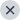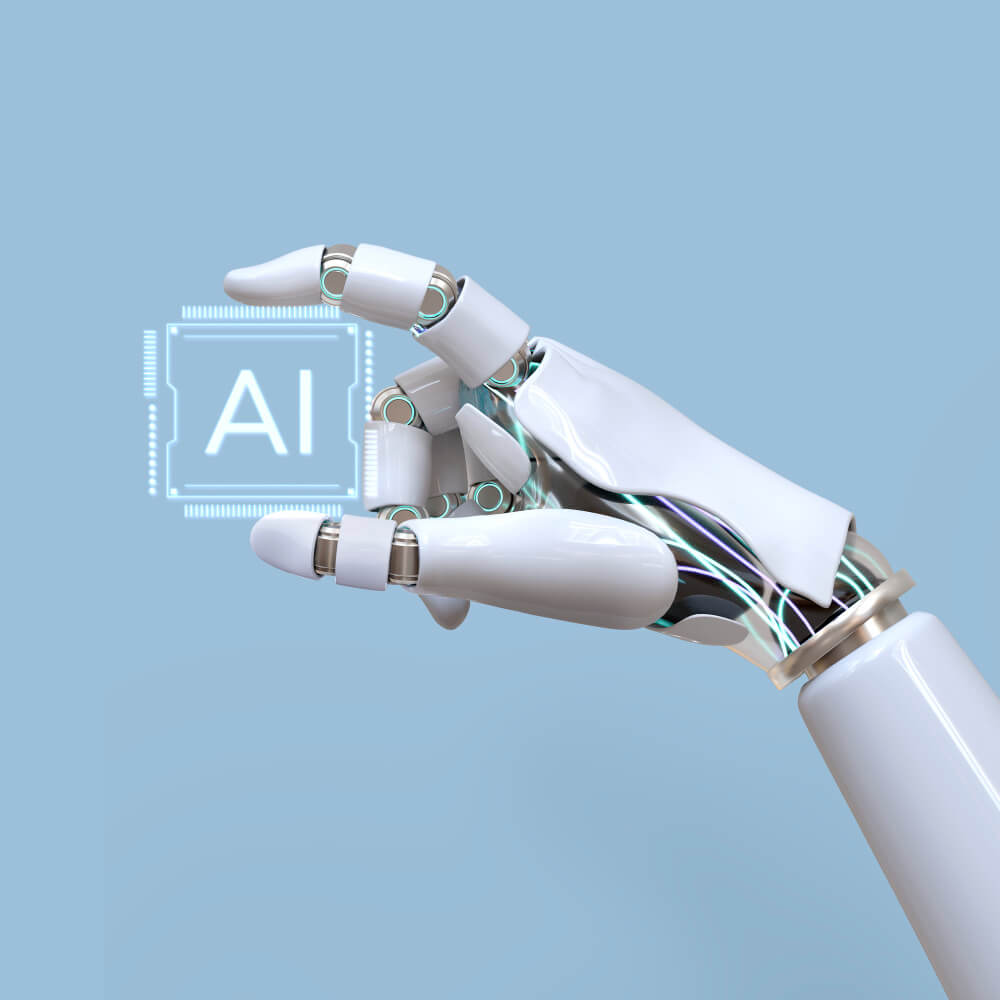0# GATE 2024 Syllabus For Data Science and Artificial Intelligence (DA)

16 August, 2023 By Prateek Mishra, IMS, GATE Mentor

Data Science and Artificial Intelligence (DA) paper was recently announced for GATE 2024, and IISc Bangalore officially released it in their brochure. The GATE syllabus for Data Science and Artificial Intelligence (DA) 2024 covers a wide range of topics like Probability, statistics, linear algebra,  algorithms, Programming, Data Structures, database management systems, warehousing, machine learning and Artificial intelligence.

## Eligibility

Candidates from engineering branches like Computer science, Electronics and Telecommunication, Electrical, Mechanical and Engineering science are eligible to write the DA paper.
Also, Mathematics, Physics, and Statistics candidates are eligible to write the DA paper.

## GATE Syllabus for Data Science and Artificial Intelligence (DA) 2024

GATE Syllabus For Data Science and Artificial Intelligence (DA) 2024 is designed to measure a candidate’s knowledge of statistics, analytics, data structures and coding through Python.  Moreover, aspirants must thoroughly go through and understand the GATE syllabus before starting the preparation for the DA paper.

Below is the GATE DA Syllabus in the table below:

 Probability and Statistics Counting (permutation and combinations), probability axioms, Sample space, events, independent events, mutually exclusive events, marginal, conditional and joint probability, Bayes Theorem, conditional expectation and variance, mean, median, mode and standard deviation, correlation, and covariance, random variables, discrete random variables and probability mass functions, uniform, Bernoulli, binomial distribution, Continuous random variables and probability distribution function, uniform, exponential, Poisson, normal, standard normal, t-distribution, chi-squared distributions, cumulative distribution function, Conditional PDF, Central limit theorem, confidence interval, z-test, t-test, chi-squared test. Linear Algebra . Vector space, subspaces, linear dependence and independence of vectors, matrices, projection matrix, orthogonal matrix, idempotent matrix, partition matrix and their properties, quadratic forms, systems of linear equations and solutions; Gaussian elimination, eigenvalues and eigenvectors, determinant, rank, nullity, projections, LU decomposition, singular value decomposition. Calculus and Optimization Functions of a single variable, limit, continuity and differentiability, Taylor series, maxima and minima, optimisation involving a single variable. Programming, Data Structures and Algorithms Programming in Python, basic data structures: stacks, queues, linked lists, trees, hash tables; Search algorithms: linear search and binary search, basic sorting algorithms: selection sort, bubble sort and insertion sort; divide and conquer: merge sort, quicksort; introduction to graph theory; basic graph algorithms: traversals and shortest path. Database Management and Warehousing ER-model, relational model: relational algebra, tuple calculus, SQL, integrity constraints, normal form, file organisation, indexing, data types, data transformation such as normalisation, discretisation, sampling, compression; data warehouse modelling: schema for multidimensional data models, concept hierarchies, measures: categorisation and computations. Machine Learning Supervised Learning: regression and classification problems, simple linear regression, multiple linear regression, ridge regression, logistic regression, k-nearest neighbour, naive Bayes classifier, linear discriminant analysis, support vector machine, decision trees, bias-variance trade-off, cross-validation methods such as leave-one-out (LOO) cross-validation, k-folds cross-validation, multi-layer perceptron, feed-forward neural network;  Unsupervised Learning: clustering algorithms, k-means/k-medoid, hierarchical clustering, top-down, bottom-up: single-linkage, multiple linkages, dimensionality reduction, principal component analysis. AI Search: informed, uninformed, adversarial; logic, propositional, predicate; reasoning under uncertainty topics – conditional independence representation, exact inference through variable elimination, and approximate inference through sampling

The Data Science and AI GATE exam is not just an examination; it’s a gateway to a future powered by innovation, insight, and intelligence. The rewards are abundant for engineering graduates who dare to step into this dynamic world – from lucrative career prospects to the thrill of shaping the future. So, if you’re an engineering graduate with a thirst for knowledge and a passion for technology, consider taking the Data Science and AI GATE exam – your pathway to unlocking a world of limitless opportunities.